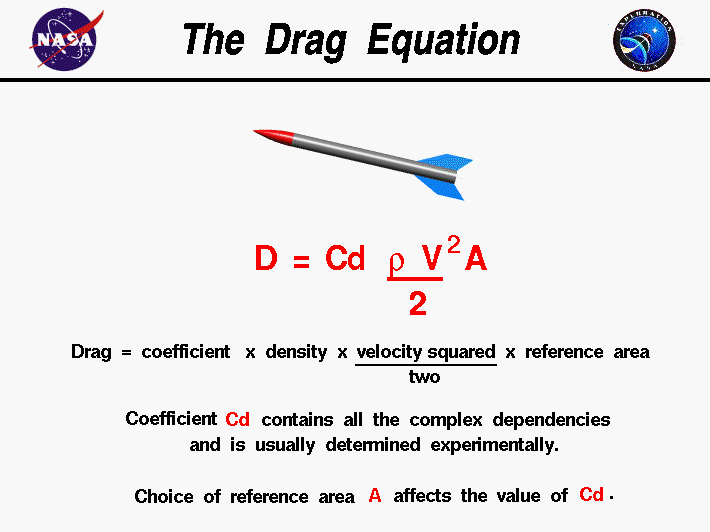# How is drag applied to force?

How does a rigidbody's drag value work with force? Let's say a force of 5 is added to an object which has a drag of 1. ForceMode.Force in FixedUpdate().

I'm interested in the formula used to determine the velocity. Thanks!

A guess: velocity.y - ( drag * (fixed time step/10) ) That seems to match up... but is it right? The division is because it seems to be one decimal place off compared to what I see in practice, so I think I'm missing a piece.

I haven’t got the exact formula used by the Unity’s physics engine, but my investigation of its behavior gave me the answer I was looking for. Given velocity (as Vector3) and drag (as float), the drag force is applied in Unity in the way descibed by the folliwing formula:

``````float multiplier = 1.0f - drag * Time.fixedDeltaTime;
if (multiplier < 0.0f) multiplier = 0.0f;
velocity = multiplier * velocity;
``````

How have I got it? Well, I wrote the script which computed the expected value of velocity’s change (using this formula) at every fixed frame. I also wrote an another script measuring the value of the real velocity’s change. I attached the both scripts to an object placed in the air. I also attached the rigidbody component to the object and disabled its gravity. Then during gameplay I gave a single forward impulse to the object’s rigidbody. Both expected and real values of velocity’s change were shown on the screen and updated every fixed frame, and they were always the same.

It’s interesting that according to the abovementioned formula a rigidbody will be stopped immediately if its drag equals the number of fixed updates per second. For example, if we have 50 fixed updates per second, the drag being set to 50 or greater will prevent its rigidbody from moving.

I should also notice that in the real life the force of airdrag is non-linear and changes proportionally with the square of an object’s velocity. Therefore, the Unity’s formula is very rough. If you’re making the game with the airdrag being the key factor of gameplay, you should set a rigidbody’s drag to zero and apply the drag in your own script using the more accurate formula.

This is the formula I used:

``````Vector3 drag_vel = wind_vel - vel;
Vector3 drag_accel = drag_vel * drag_vel.magnitude * 0.5f * air_density * drag_coeff * ball_cross_section / ball_mass;

...

return gravitational_accel + magnus_accel + drag_accel;
``````

According to NASA, Drag is related with Velocity^2, so the Drag number in Unity can be an approximation of the rest of this formula because according to this, in reality this number is not even constant because the Area towards the Velocity can change, and unity doesn’t care about the shape of the objectNASA - Drag Formula